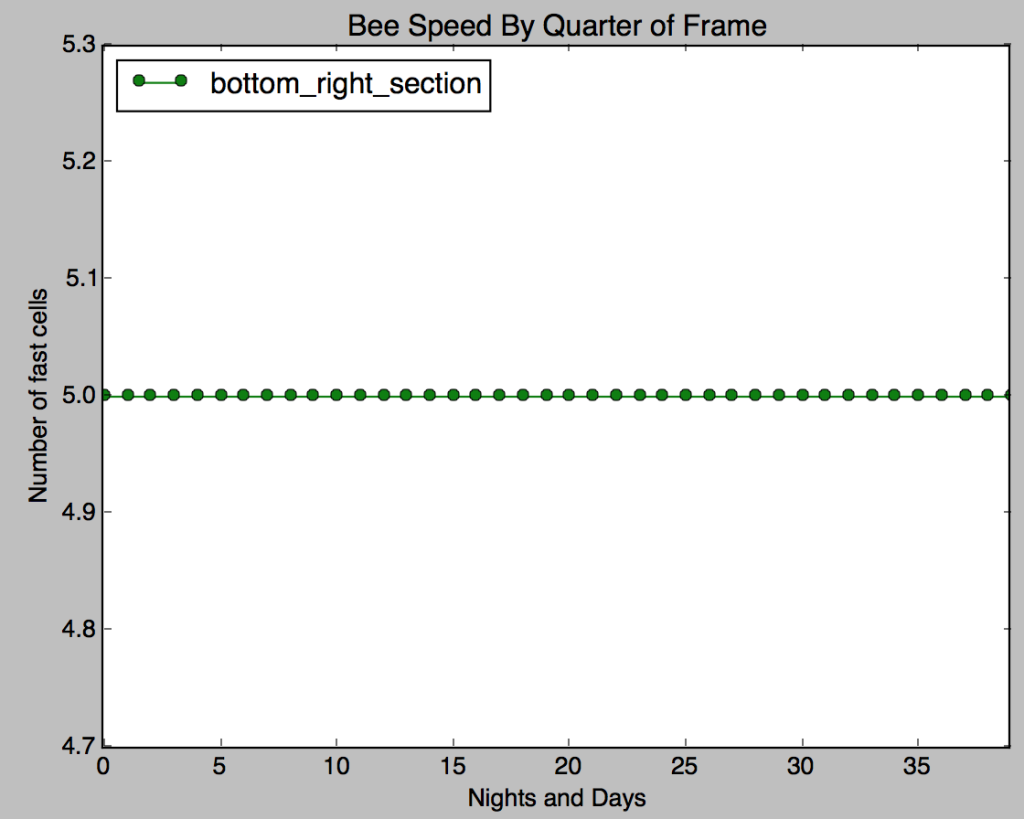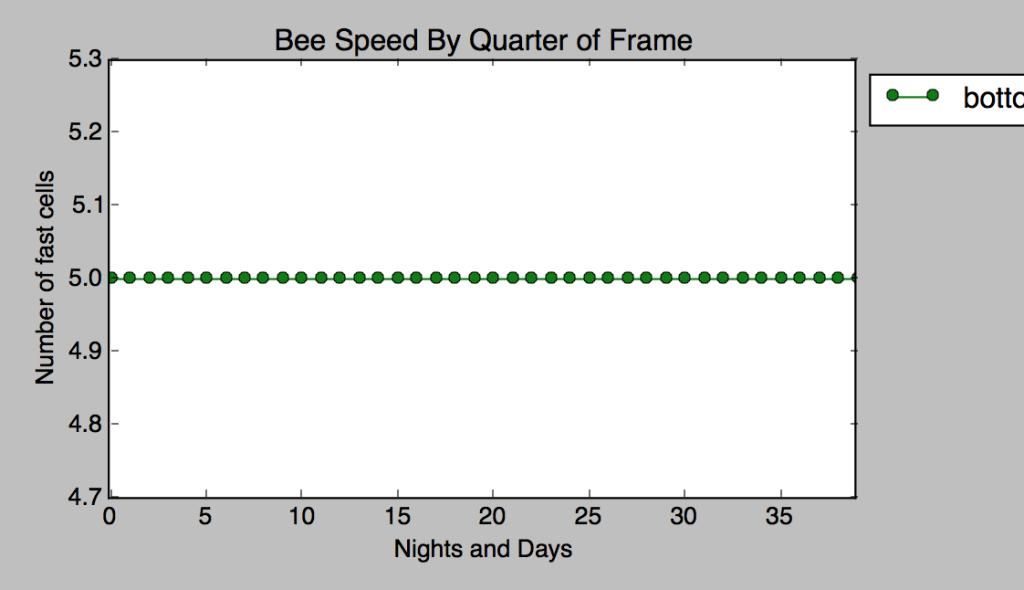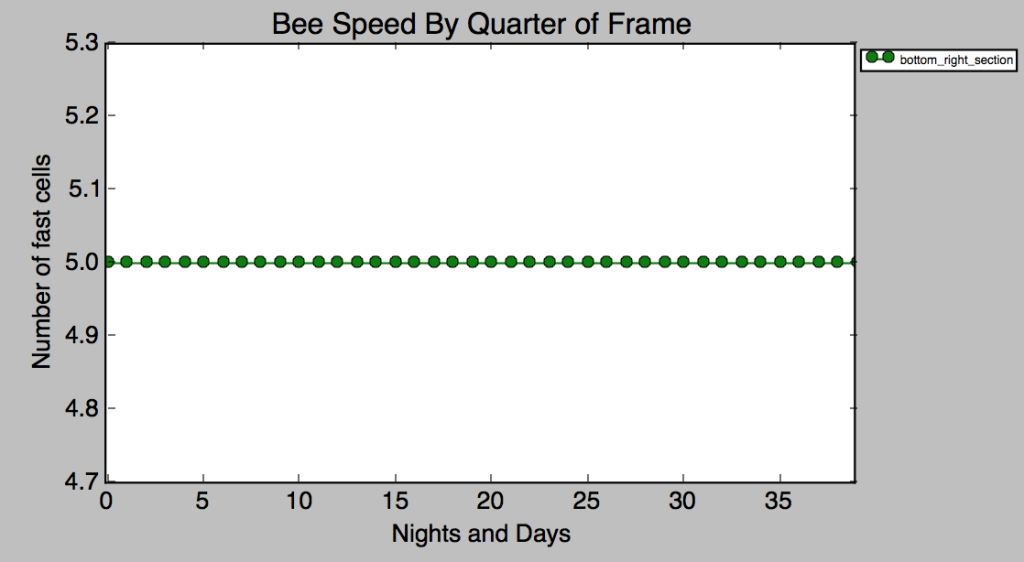Positioning a legend outside the figure with Matplotlib and Python

One of the things that has been a little frustrating lately has been what to do if you need a legend for your plot, yet there’s so much content on your plot you need to place it next to the figure, rather than within it.  The standard way to create a plot with the legend within it looks like this:```import matplotlib.pyplot as plt
import numpy as np

plt.figure()
plt.plot([5,5,5,5,5,5,5,5,5,5,5,5,5,5,5,5,5,5,5,5,5,5,5,5,5,5,5,5,5,5,5,5,5,5,5,5,5,5,5,5], '-go', label='bottom_right_section')
plt.xlim([0,39])
plt.title('Bee Speed By Quarter of Frame')
plt.xlabel("Nights and Days")
plt.ylabel("Number of fast cells")
plt.legend(loc='upper left')
plt.show()
```

The first step I tried to move the legend outside the figure was to add this code to the “legend” method:

`plt.legend(loc='upper left', bbox_to_anchor=(1,1)) `Unfortunately, the legend was being cut-off on the right hand side. I then tried to shrink down the legend (as it was rather large) and when that didn’t work, I found out that I could pass a padding argument to the “tight_layout” method which finally solved the issue:

```plt.legend(loc='upper left', prop={'size':6}, bbox_to_anchor=(1,1))
```The final code to solve the problem with establishing a large margin around the figure:

```
import matplotlib.pyplot as plt
import numpy as np

plt.figure()
plt.plot([5,5,5,5,5,5,5,5,5,5,5,5,5,5,5,5,5,5,5,5,5,5,5,5,5,5,5,5,5,5,5,5,5,5,5,5,5,5,5,5], '-go', label='bottom_right_section')
plt.xlim([0,39])
plt.title('Bee Speed By Quarter of Frame')
plt.xlabel("Nights and Days")
plt.ylabel("Number of fast cells")
plt.legend(loc='upper left', prop={'size':6}, bbox_to_anchor=(1,1))
plt.show()

```
The following two tabs change content below.Jack Simpson

Computational biology PhD candidate at the Australian National University. I love writing (both articles and software), learning more about the world around us, and beekeeping. I also write for BioSky.co